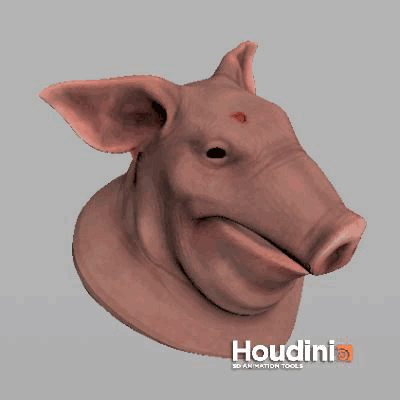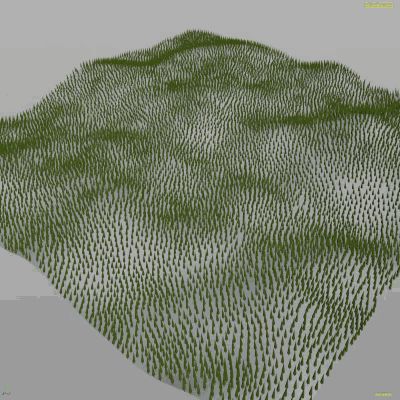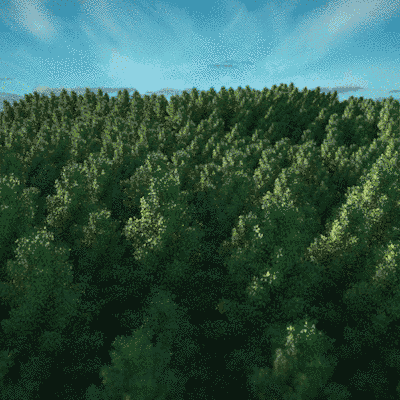My life has improved with the wrangle SOP and VEX. I don't know why i did shy away from that the first years I used Houdini, probably because it can look a bit intimidating. As a matter of fact, it is often actually more straightforward as the SOP equivalents. It's especially useful inside a solver sop, too.

##### Global / external attributes:
``````@Frame //current frame
@Time //current time, second-wise
@ptnum //this point's id
@primnum //this prim's id
@numprim //total prim number
@numpt //total point number

@P //point position
@N //normal
``````
##### Setting attributes
``````//will just create a float attribute.
@myattrib;
//vector attribute
v@vectValue = {1,1,1};
//float attribute
f@floatValue = 0.2;
//int attribute
i@intValue = 2;
``````
##### Get user input

Believe it or not, Houdini will auto-create user input for you in a wrangle SOP:

``````//Create a float slider mapped to myfloat
f@myfloat = ch('float');
//Same with a vector
v@myvec = chv('vec');
//Int
i@myint = chi('int');
//Float ramp. Grab the value at the position ramppos.
float ramppos = 0.5;
f@otherfloat = chramp('ramp', ramppos);
``````
##### Some snippets

Set point attribute by point id. Useful in loops.

``````setpointattrib(0, "attribute", @ptnum, val);
``````

Refer to geometry in the second input

``````point(@OpInput2, "Cd", @ptnum);
``````
##### Randomize normal direction
``````v@N = normalize(@N + (ch('random_factor')* sample_direction_uniform(random(@P))));
``````
##### Blur attribute
``````int pchandle = pcopen(0, 'P', @P, 1, chi('pts_to_average'));
@attrib = pcfilter(pchandle, 'attrib');
``````
##### Iterate through prim's verts
``````for (int i=0; i < primvertexcount(0, @primnum) ; i++) {
vector myP = vertex(0, "P", i);
}
``````
##### Poor man's uvlayout

Following an uvtexture's face option, you might want to 'fit' uvs proportionally into 0-1 space. You could use uvlayout but that might rotate or such and is very slow depending on your setup.

``````vector minP = {100000, 100000, 100000};
vector maxP = {-100000, -100000, -100000};

// iterate once, get extents
for (int i=0; i < primvertexcount(0, @primnum) ; i++) {
vector currentUV = vertex(0, "uv", @primnum, i);
minP.x = min(minP.x, currentUV.x);
maxP.x = max(maxP.x, currentUV.x);
minP.y = min(minP.y, currentUV.y);
maxP.y = max(maxP.y, currentUV.y);
}

// calculate sizes
float xSize = maxP.x - minP.x;
float ySize = maxP.y - minP.y;
float largestSize = max(ySize, xSize);

for (int i=0; i < primvertexcount(0, @primnum) ; i++) {
vector origUV = vertex(0, "uv", @primnum, i);
// transform to 0,0
vector correctedUV = set(origUV.x + (minP.x*-1), origUV.y + (minP.y*-1), 0);
// stretch / shrink to bounds
correctedUV.x *= 1/largestSize;
correctedUV.y *= 1/largestSize;
// set the thing
setvertexattrib(0, "uv", @primnum, i, correctedUV);
}

``````
``````vector scale = {1,1,1};
scale = scale * rand(@P);

matrix3 m_trans = primintrinsic(0, "transform", @ptnum);
matrix scalem = maketransform(0, 0, {0,0,0}, {0,0,0}, scale, @P);
m_trans *= matrix3(scalem);

//overall randomness: 20 deg around Y
rotate(m_trans, roat_rand, {0,1,0});
setprimintrinsic(0, "transform", @ptnum, m_trans);
``````
##### Create points at primitive centers (primitive wrangle)
``````int pt = addpoint(0, @P);
removeprim(0, @primnum, 1);
setpointattrib(0, "N", pt, @N);
``````
##### Rotate stuff:
``````@P = qrotate( quaternion( {0,90,0}, 0 ), @P );
``````
##### Some animations:

Infection-style wrangle:``````if (@tagged == 0) {
float maxdist = ch('maxdist');
int maxpoints = 8;
int pts[] = nearpoints(0,@P, maxdist, maxpoints);
int pt ;

foreach  (pt;pts) {
if (point(0,'tagged',pt)==1) {
@tagged=1;
v@Cd = {1,0,0};
@age = @Frame;
return;
}
}
}
else{
@P.y = @P.y-0.1;
}
``````Crashing trees:Each point stores a direction to the next untagged point, then vectors are interpolated

``````v@N2;
v@dir;

if (@tagged == 0) {
@N2 = @N;
float maxdist = ch('maxdist');
int maxpoints = 3;
int pts[] = nearpoints(0, @P, maxdist, maxpoints);
int pt ;

foreach  (pt;pts) {
if (point(0,'tagged', pt)==1) {
@tagged=1;
@dir = @P - point(0, 'P', pt);
@dir.y -= 0.0001;
return;
}
}
}
else{